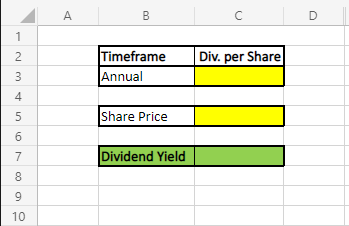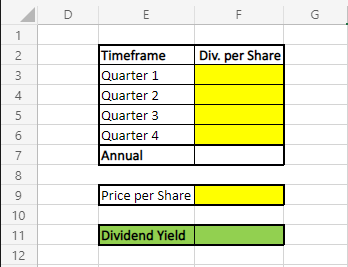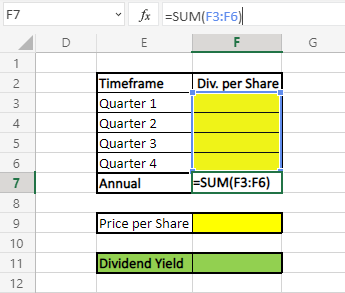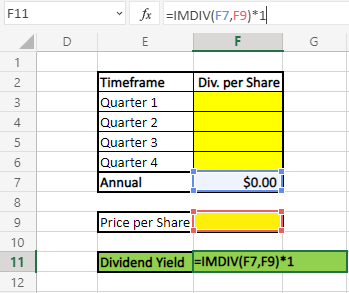# How to Calculate Dividend Yield

Companies pay out dividends each year to shareholders and investors relative to the price of its stock. These dividends are often paid by mature companies on a consistent quarterly basis, although companies have the freedom to pay out dividends to shareholders at any time.

For some investors, certain companies are targeted investments based on the consistency and yield of the dividends paid by the targeted company. This calculation helps investors determine which companies to invest in and how much taxes to expect to pay on those dividends.

This article will illustrate the dividend yield formula, how to calculate dividend yield step by step, and how to create a dividend yield calculator.

## What is Dividend Yield

### Dividend Yield Formula

Dividend yield is calculated through dividing the annual dividends per share by the price per share for a particular company’s stock then multiplying the product by 100. Data for this calculation can be gathered from the previous year’s financial report or from the last four quarters of dividends paid by the company.

DY = (Annual Dividends per Share / Price per Share) x 100

Where:

DY = Dividend Yield

## How to Calculate Dividend Yield

### Calculation Step by Step

1. Determine the price per share of a company’s stock.
2. Determine the annual dividends per share of a company’s stock.
3. Divide annual dividends per share by the price per share to solve.

Following these steps will produce the result expressed in terms of a percentage reflecting the amount of money a company distributed to shareholders for owning a share of its stock divided by the current price of that stock.

### Example

An investor is trying to determine the dividend yield for a publicly-traded financial services company in order to make a sound investment decision.

Based on information the investor has gathered, the price per share of the company is \$32.00 per share.

After reviewing the previous year’s financial report, the investor has determined the company’s annual dividend per share to be \$1.08 per share. Since the dividend paid by this company is on a quarterly basis at a consistent rate, the investor is comfortable using this figure in their dividend yield formula as they feel it is an accurate value.

Using this information, the investor will identify the yield by dividing the annual dividends per share by the price per share of this company’s stock and multiplying the product by 100:

DY = (Annual Dividends per Share / Price per Share) x 100

DY = (1.08 / 32.00) x 100

DY = (0.03375) x 100

Dividend Yield = 3.38%

Here we can see that the yield for the publicly-traded financial services company is 3.38%, which reflects the amount of money a company will pay its shareholders for owning a share of its stock divided by the price of the share.

If the investor is comparing this yield to that of another company, the investor will be more likely to purchase shares of the company with the higher yield percentage (assuming all other factors are equal).

## Dividend Yield Calculator

For investors comparing the yields of multiple companies, building a dividend yield calculator can significantly simplify the process – especially since the way companies pay dividends differs and may require different approaches.

How to calculate dividend yield using two different methods can be integrated into a calculator using Microsoft Excel.

### Dividend Calculator Method Number 1:

When the annual dividends per share can be accurately calculated from a company’s financial report, the Excel formula to calculate the yield is relatively simple.

The annual dividends per share, share price, and yield cells will be arranged as follows:After formatting the dividend calculator as shown, the following formula will be inserted into cell C7, which contains the product of the calculator:

=IMDIV(C3,C5)*1

Once the dividend yield formula has been entered into cell C7, the annual dividends per share and share price of a particular company can be entered into cells C3 and C5. Based on the earlier example of the publicly-traded financial services company, if the annual dividends per share were \$1.08 per share and the price per share was \$32.00, then the calculator would appear as the following:

### Dividend Calculator Method Number 2:

At some points, it may make more sense for an investor to add the dividends paid from the previous four quarters to calculate the annual dividends per share, rather than using the last financial report of a company. When the rate of dividends paid changes or if the financial report has become old and outdated, this method is more sufficient to provide an accurate value for annual dividends per share.

The quarterly dividends per share paid, the share price, and the yield will be arranged as follows:Once the dividend yield calculator has been arranged as shown, the following formula will be inserted into the annual dividends per share cell of F7. This formula will add the quarterly dividends paid to determine the annual dividends paid per share:

=SUM(F3:F6)Next, the following formula will be inserted into the yield cell of F11 in order to derive the dividend yield from the annual dividends per share and the price per share:

=IMDIV(F7,F9)*1Once this formula has been inserted into the yield cell, the other cells can now be populated with the relevant information. For example, if a company has a share price of \$52.00 and quarterly dividends per share of \$0.40, \$0.42, \$0.44, and \$0.46, the dividend yield calculator will show the following:

## Tips for Calculating

### Tip Number 1:

The dividend yield formula is only as accurate as the information used in the calculation. If the dividend rate has changed since a company’s last financial report, use the method of drawing dividend data from the past four quarters to calculate the annual dividends per share value. Using more recent and more similar data such as this will help you produce a yield with higher confidence and accuracy.

### Tip Number 2:

Dividend yield represents the rate of return of cash dividends paid to shareholders over the course of a year. This is not the same as the dividend payout ratio, which illustrates how much of a particular company’s net earnings are eventually paid out as dividends to shareholders. The two are very different indicators and should be recognized accordingly. The dividend yield only takes into consideration the annual dividends per share and the price per share.

### Tip Number 3:

After calculating the dividend yield of a company, compare the company’s yield to the yield average of the industry that company is a part of. The yield average varies by industry. For example, real estate companies and real estate investment trusts (REITs) have higher average yields, whereas companies specializing in various technologies return lower average yields.

## Takeaways

For those wondering “What is dividend yield?”, this type of yield is calculated by dividing the annual dividends per share by the price per share of a particular company. This popular metric represents the amount of cash paid to a shareholder for owning one share of a company’s stock, divided by the price of that share.

For investors comparing different companies to invest in, comparing various yields is helpful to making a sound investment decision, as some companies pay higher dividends more often than others. When calculating this value, make sure the annual dividends per share is accurately calculated to produce a reliable result.

### Surface-enhanced Raman spectroscopy

Surface-enhanced Raman spectroscopy gives the same information as normal Raman spectroscopy but with a significantly enhanced signal. It is also referred to as surface-enhanced Raman

### Mössbauer Spectroscopy

In 1958, a German physicist, Rudolf Mossbauer, invented Mossbauer spectroscopy. It is a versatile technique that provides sensitive information about the structural, magnetic, chemical and

### Energy dispersive spectroscopy (EDS)

Energy dispersive spectroscopy (EDS) is an interesting analytical spectroscopic technique. It is mainly used to characterize and find the relative abundance of different chemical elements